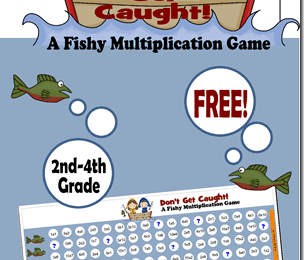Category - Multiplication

Make learning math fun with our HUGE variety of free multplication printables! These fun, hands-on multiplication activities, multiplication worksheets, and multiplicatin games are sure to make practicing fun for kids of all ages. These are great for parents, teachers and homeschoolers to add extra math practice to help students gain math fluency in grade 3 math, grade 4 math, grade 5 math, and grade 6 math.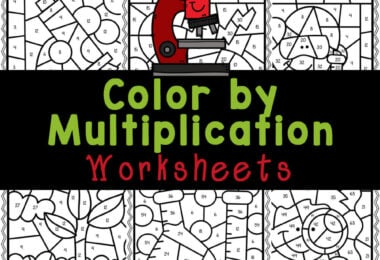Science Color By Multiplication Worksheets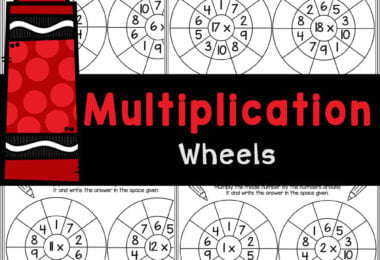FREE Printable Multiplication Wheels Worksheets Activity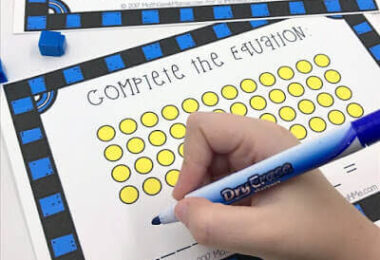FREE Printable Interactive Multiplication Mats for Teaching Kids...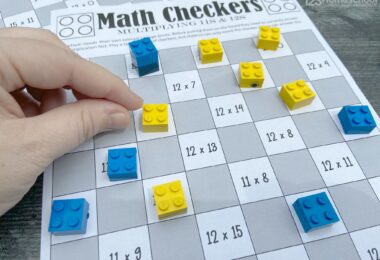FREE Multiplication Checkers Printable Math Game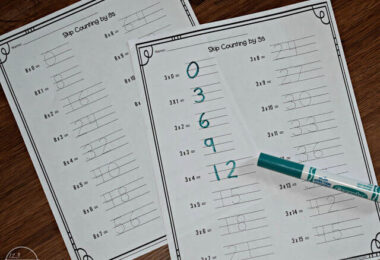Skip Counting Worksheets (1-15s)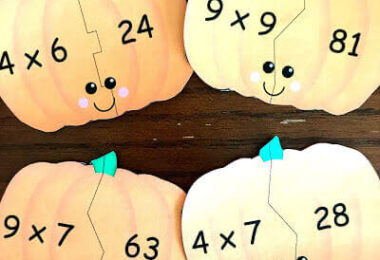Pumpkin Multiplication Puzzle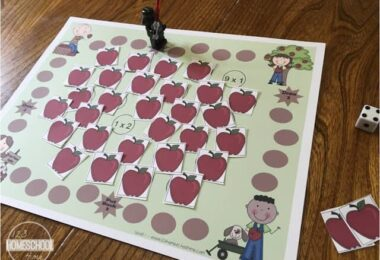Apple Math Printable Multiplication Game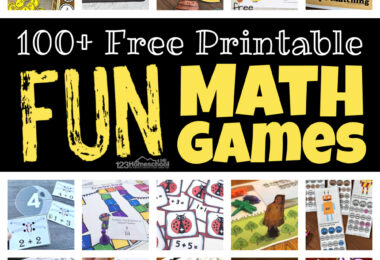FUN Printable Math Games for Elementary Age Kids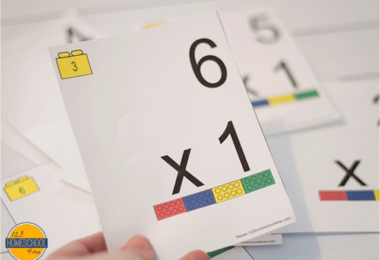Lego Printable Multiplication Flash cards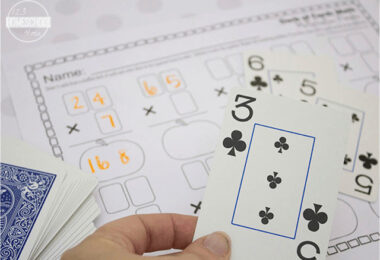Free Printable Multiplication Worksheets with a Deck of Cards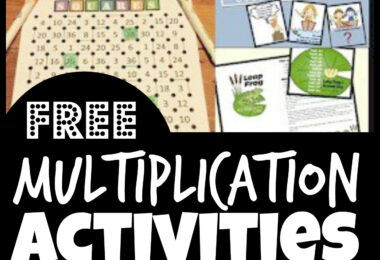FREE Printable Multiplication Games and Activities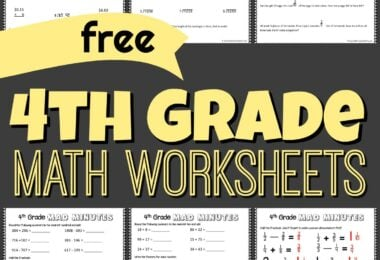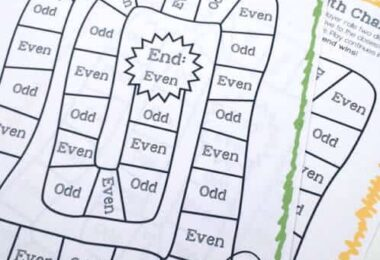Free Printable Summer Math Games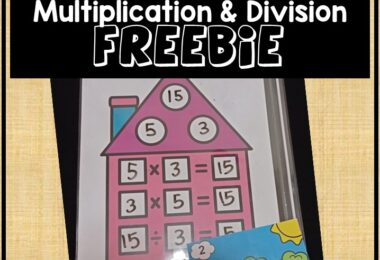Multiplication and Division Fact Family Task Cards &...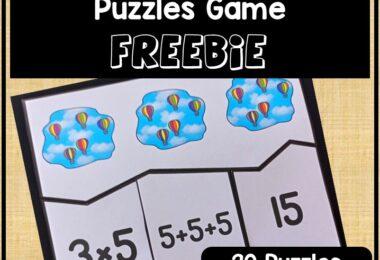Hands-On Multiplication Puzzles Activity – FREE Printable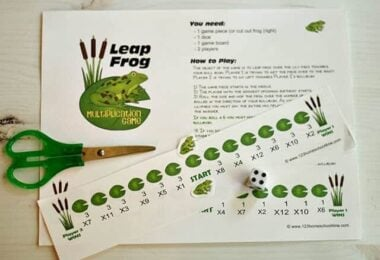Leapfrog Printable Multiplication Game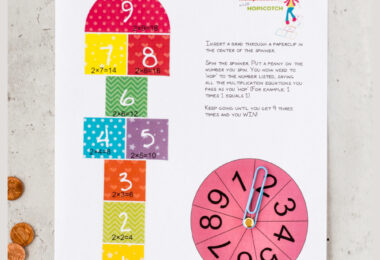Multiplication Hopscotch Printable Math Game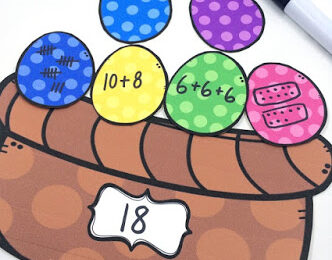Easter Basket Math Challenge w/ FREE PrintablesSpring Multiplication Math Craft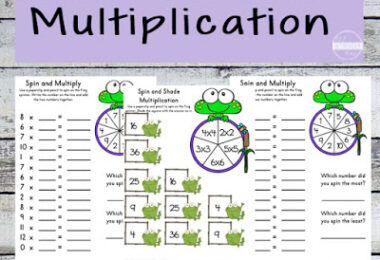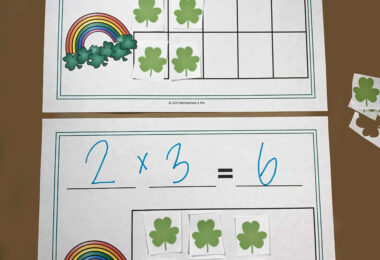FREE St Patrick’s Day Math Equation Mats Printable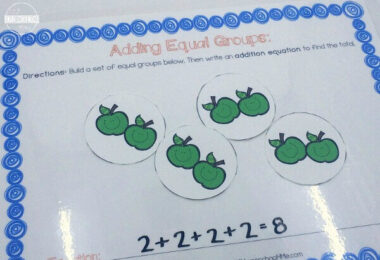Hands-On Introduction to Multiplication Printable Activity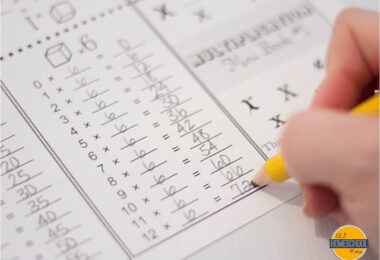Free Multiplication Worksheets —> Multiplication Booklet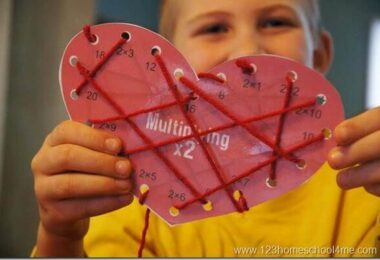FREE Printable Multiplication Valentines Day Math Activity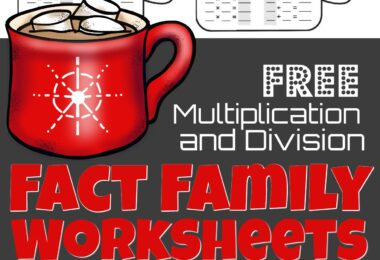Winter Hot Cocoa Multiplication and Division Math Worksheets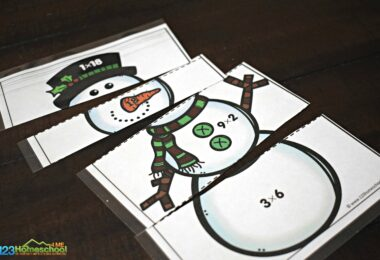FREE Printable Snowman Math Multiplication Puzzles for Winter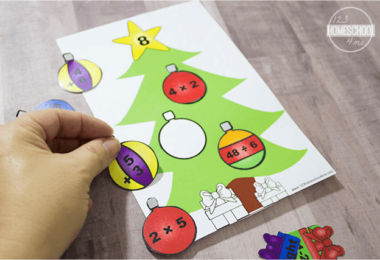Decorate the Math Christmas Trees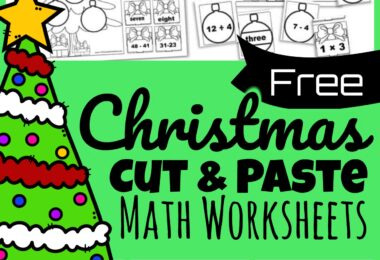Christmas Math Worksheets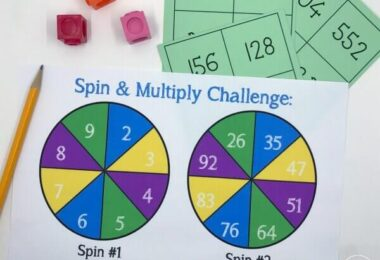Multiplication Spinner Game – Spin and Multiply!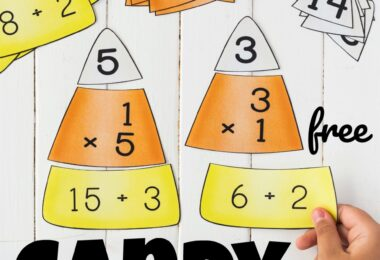Candy Corn Math – Multiplication and Division Puzzles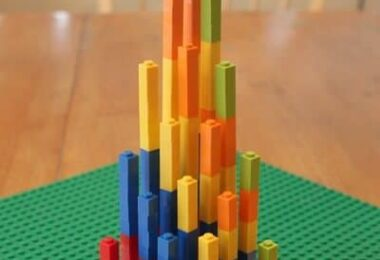LEGO Multiplication Math Activity